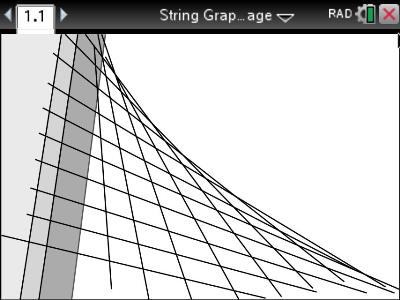# Activities

•• ##### Subject Area

• Standard: VCE: Specialist Mathematics: Specialist Mathematics

• ##### Author45 Minutes

• ##### Software

TI-Nspire™ CAS

4.4

## Advanced String Graphs Part 1#### Activity Overview

The famous designs of Santiago Calatrava represent beautiful examples of the synergy between mathematics, engineering and architecture. In this activity students model the cables of the “Bridge of Stings” in Jerusalem using a family of straight lines. The envelope formed by these straight lines can also be modelled by a single equation defining the curve. Students determine equations to straight lines, solve simultaneous equations, generate parametric equations and finally a single equation to model the resulting curve.

#### Objectives

• Cartesian and parametric forms of lines and parabolas
• Solutions to simultaneous equations
• Generalisation of families of lines using parameters

#### Vocabulary

• Parameter
• Simultaneous equations
• Envelope